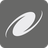Black Holes
Simple+
English

"Wow! That was amazing!" Stephen was greatly impressed. He took his eyes off the telescope and leaned back.

"Did you see that?" asked Sofia.

...moments ago a super massive black hole had just "swallowed" another one...

"You were right Sofia, that was an incredible event" Stephen said. "So, can I join your A&A (Astronomy & Astrophysics) research group?"

Sofia looked at him triumphantly and said - "Really, my plan was to rope you in anyway! We are currently researching black holes and their gravitational fields and we are looking for someone to help us create a software model that will predict the future state of black holes".

"Hmm, it's very interesting. Let me try...".

You need to help Stephen implement a software model (function) that predicts the state of black holes under a controlled environment. The A&A research team has identified some peculiarities in the behavior of black holes.

To create the software model one should take into account:

1. The cartesian coordinate system is used to map out the black holes.
2. Each black hole is represented as a circle with x, y (center coordinates) and r (radius).
3. In contrast to the area, which may change during the absorption process, the coordinates remain constant.
4. The area of a black hole greatly influences its mass, and consequently, the gravitational field.
5. The absorption order of black holes depends on the distance between their centers, starting with the black holes that are closest to each other. If the distance between different black holes is equal, then the leftmost black hole in the list should merge first.
6. The absorption process (merging) of black holes is possible if and only if the following conditions are met:
- The intersection area of the two black holes is greater than or equal to 55% (>= 55%) of one of the two black holes area.
- The area of one of the two black holes is over 20% (>= 20%) more than the area of the other.
7. If one black hole absorbs another, their areas are summarized as (Stotal = S1 + S2).
8. The absorption process continues as long as all conditions are met (see p. 6).

Let's look at some simple examples:

 Input: [(2, 4, 2), (3, 9, 3)] No intersection Output: [(2, 4, 2), (3, 9, 3)]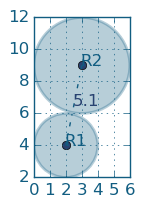Input: [(0, 0, 2), (-1, 1, 2)] S1 = 12.57; S2 = 12.57 Intersection area = 7.03 p1 = 56% p2 = 56% Domination: 0% Output: [(0, 0, 2), (-1, 1, 2)]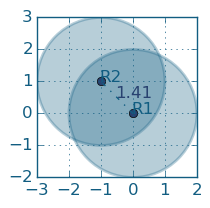Input: [(1, 3, 2), (1, 3, 2.19)] S1 = 12.57; S2 = 15.07 Domination: 19.9% Output: [(1, 3, 2), (1, 3, 2.19)]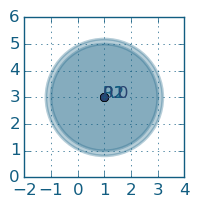More complex example: [(2, 2, 3), (0, 4, 2), (4, 6, 2), (4.7, 3, 0.5)]

...
Input: [(2, 2, 3), (0, 4, 2), (4, 6, 2), (4.7, 3, 0.5)]
R1, R2 - distance (2.83); S1 = 28.27; S2 = 12.57
Intersection area = 6.04
p1 = 21% p2 = 48%
Output: [(2, 2, 3), (0, 4, 2), (4, 6, 2), (4.7, 3, 0.5)]
----------------------------------------------------------
Input: [(2, 2, 3), (0, 4, 2), (4, 6, 2), (4.7, 3, 0.5)]
R1, R4 - distance (2.88); S1 = 28.27; S4 = 0.79
Intersection area = 0.5
p1 = 2% p4 = 63%
Output: [(2, 2, 3.04), (0, 4, 2), (4, 6, 2)]
----------------------------------------------------------
Input: [(2, 2, 3.04), (0, 4, 2), (4, 6, 2)]
R1, R2 - distance (2.83); S1 = 29.03; S2 = 12.57
Intersection area = 6.21
p1 = 21% p4 = 49%
Output: [(2, 2, 3.04), (0, 4, 2), (4, 6, 2)]
----------------------------------------------------------
Input: [(2, 2, 3.04), (0, 4, 2), (4, 6, 2)]
R1, R3 - distance (4.47); S1 = 29.03; S2 = 12.57
Intersection area = 0.87
p1 = 3% p4 = 7%
Output: [(2, 2, 3.04), (0, 4, 2), (4, 6, 2)]
----------------------------------------------------------
Input: [(2, 2, 3.04), (0, 4, 2), (4, 6, 2)]
R2, R3 - distance (4.47)
S1 = 12.57; S2 = 12.57
No intersection
----------------------------------------------------------
Output: [(2, 2, 3.04), (0, 4, 2), (4, 6, 2)]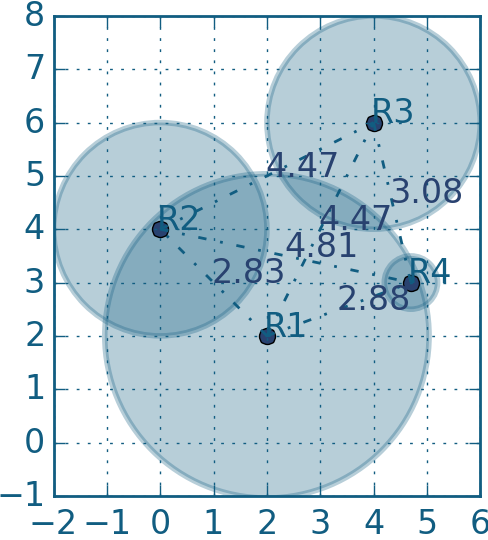After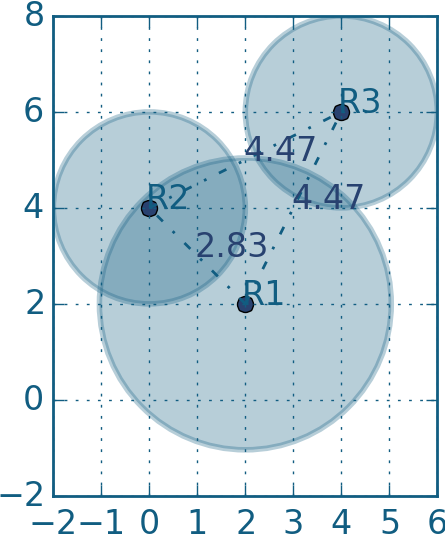You should be an authorized user in order to see the full description and start solving this mission.